# Even and Odd Numbers

Even numbers are integers that are divisible by 2. When they are divided by 2, there is no remainder.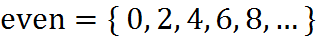Odd numbers are integers that are not divisible by 2.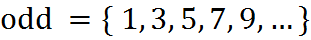Further key facts:

• Negative numbers are also included in both groups.
• The number 0 is even. Zero, when divided by 2, has no remainder (just like 2, 4, and so on).
• On the GMAT, an unknown number is not necessarily even or odd; it might be a non-integer, such as 1.4. However, if we know that a number is an integer, then it must be even or odd.

The following properties are critical for some GMAT questions: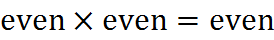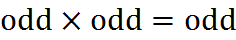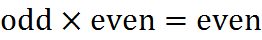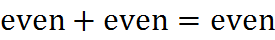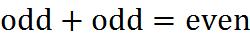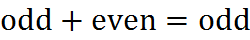You can bring these properties to mind by testing a couple of possible numbers. A conversation in your head might go like this: “What’s odd times even? Well, 3 times 2 is 6, and that’s even. So, odd times even is always even.” You’re guaranteed to see a question with odd/even rules on the GMAT… so be ready to summon a dialogue like that one into your mind.

Here is the logic behind the multiplication rules: an even number is divisible by 2, so that means it has 2 as a factor. And when two integers are multiplied, their product has all of the factors of both numbers: the factors accumulate. So if either of the two integers has a factor of 2 – if either is even – then their product is even.

Here is the logic behind the addition rules: an even number can be thought of as a bunch of pairs. For example, 6 is three pairs, and 8 is four pairs. If you add two even numbers, you still have a bunch of pairs, so the sum is even. An odd number is like a bunch of pairs with one left over. So if you add two odd numbers, you have a bunch of pairs and the two lonely units make a final pair, and the sum of two odd numbers is even. Meanwhile, if you add an even integer and an odd integer, the sum still has the unpaired unit from the odd number, so the sum is odd.

## Practice Questions

Cannot Be Odd:
http://www.gmatfree.com/Cannot-Be-Odd

Even and Odd Properties of Algebraic Expression:
http://www.gmatfree.com/Even-and-Odd-Properties-of-Algebraic-Expression

Welcome! You are encouraged to register with the site and login (for free). When you register, you support the site and your question history is saved.